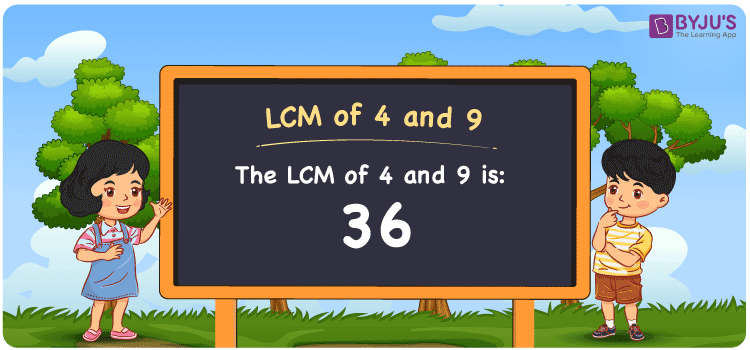Checkout JEE MAINS 2022 Question Paper Analysis : Checkout JEE MAINS 2022 Question Paper Analysis :

# LCM of 4 and 9

LCM of 4 and 9 is 36. The number which is evenly divisible by the two numbers, 4 and 9 gives you the LCM value. Least common multiples of 4 and 9 can be obtained from the common multiples. (4, 8, 12, 16, 20, 24, ….) and (9, 18, 27, 36, 45, 54,….) are the multiples of 4 and 9. The LCM of two numbers using prime factorization, division and by listing the multiples are given here for a good conceptual knowledge.

## What is LCM of 4 and 9?

The answer to this question is 36. The LCM of 4 and 9 using various methods is shown in this article for your reference. The LCM of two non-zero integers, 4 and 9, is the smallest positive integer 36 which is divisible by both 4 and 9 with no remainder.## How to Find LCM of 4 and 9?

LCM of 4 and 9 can be found using three methods:

• Prime Factorisation
• Division method
• Listing the multiples

### LCM of 4 and 9 Using Prime Factorisation Method

The prime factorisation of 4 and 9, respectively, is given by:

4 = 2 x 2 = 2²

9 = 3 x 3 = 3²

LCM (4, 9) = 36

### LCM of 4 and 9 Using Division Method

We’ll divide the numbers (4, 9) by their prime factors to get the LCM of 4 and 9 using the division method (preferably common). The LCM of 4 and 9 is calculated by multiplying these divisors.

 2 4 9 2 2 9 3 1 9 3 1 3 1 1

No further division can be done.

Hence, LCM (4, 9) = 36

### LCM of 4 and 9 Using Listing the Multiples

To calculate the LCM of 4 and 9 by listing out the common multiples, list the multiples as shown below

 Multiples of 4 Multiples of 9 4 9 8 18 12 27 16 36 20 45 24 54 28 36 40

LCM (4, 9) = 36

## Video Lesson on Applications of LCM## LCM of 4 and 9 Solved Examples

What is the smallest number exactly divisible by 4 and 9?

Solution:

We know that

The smallest number which is exactly divisible by 4 and 9 is the LCM.

Multiples of 4 = 4, 8, 12, 16, 20, 24, 28, 32, 36, …..

Multiples of 9 = 9, 18, 27, 36, 45, 54, ….

Hence, the LCM of 4 and 9 is 36.

## Frequently Asked Questions on LCM of 4 and 9

### Calculate the LCM of 4 and 9 using the prime factorization method.

To determine the LCM using the prime factorization, we first have to find the prime factors.

4 = 2 x 2

9 = 3 x 3

LCM of 4 and 9 is the product of prime factors raised to the highest exponent among the numbers 4 and 9

LCM of 4 and 9 = 2² x 3² = 36

### If the LCM of 4 and 9 is 36, find the GCF.

LCM x GCF = 4 x 9

Given

LCM of 4 and 9 = 36

36 x GCF = 36

GCF = 36/36 = 1

### What is the relation between GCF and LCM of 4 and 9?

The relation between GCF and LCM of 4 and 9 is

LCM x GCF = 4 x 9

LCM x GCF = 36